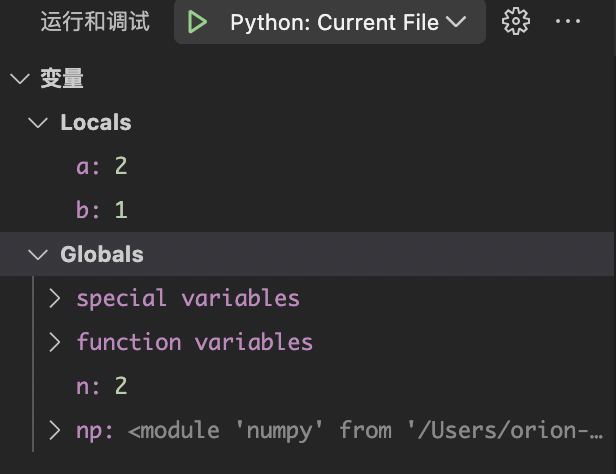# 1 local和global变量

import numpy as np
n = 2
def func(a):
b = 1
return a + b

print(func(n)) # 3n = 2
def func(a):
b = 1
return a + b + n # 对全局变量进行读取

print(func(n)) # 5


n = 2
def func(a):
n += 1 # 对全局变量进行修改
b = 1
return a + b + n

print(func(n))


UnboundLocalError: local variable 'n' referenced before assignment


n = 2
def func(a):
global n
n += 1
b = 1
return a + b + n

print(func(n)) # 6


# 2 nonlocal 变量

# outside function
def outer():
message = 'local'

# nested function
def inner():

# declare nonlocal variable
nonlocal message

message = 'nonlocal'
print("inner:", message)

inner()
print("outer:", message)

outer()


inner: nonlocal
outer: nonlocal


# outside function
def outer():
message = 'local'

# nested function
def inner():

# declare global variable
global message

message = 'nonlocal'
print("inner:", message)

inner()
print("outer:", message)

outer()


inner: nonlocal
outer: local


def sample():
n = 0
# 闭包函数
def func():
nonlocal n
n += 1
print("n =", n)
return func

f = sample()
f() # n = 1
f() # n = 2


posted @ 2022-11-27 01:01  orion-orion  阅读(39)  评论(0编辑  收藏  举报